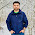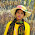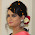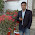## 10.12.14

### write a c++ program which contains a constructor to make sum of all multipliers of 7 or 9 between 1 and 100.

#include<iostream>
using namespace std;
class divis
{
public:
int sum=0;
divis();
~divis();
void result();
};

divis :: divis()
{
int a=1;
while(a<100)
{
if ( (a%7==0) || (a%9==0) )
{
cout << a <<"\n";
sum = sum+a;
}
a++;
}
}

divis :: ~divis()
{
cout <<"memory released" << "\n";
}

void divis :: result()
{
cout << "\n The sum of all divisibles: "<< sum << endl;
}

int main( )
{
divis vs;
vs.result();
}

#### 6 comments:

1.This comment has been removed by the author.

2.#include
using namespace std;
class divis
{
public:
divis();
~divis();
void result();
};
divis::divis()
{
cout<<"constructing";
}
divis::~divis()
{
cout<<"destructing";
}
void divis::result()
{
int sum=0,a=1;
while(a<100)
{
if ((a%7==0)||(a%9==0))
{
cout<<a<<"\n";
sum=sum+a;
}
a++;
}
cout<<"\nThe sum of all divisibles: "<<sum<<endl;

}
int main()
{
divis vs;
vs.result();
}

3.#include
using namespace std;
class multipliers
{
public:
int sum=0,number;
multipliers();
~multipliers();
void getsum();
};
multipliers::multipliers()
{
}
multipliers::~multipliers()
{
}
void multipliers::getsum()
{
cout<<"All multipliers of 7 or 9 between 1 and 100 is : ";
for(number=1;number<=100;number++)
{
if ((number%7==0)||(number%9==0))
{
cout<<number<<" ";
sum+=number;
}
}
cout<<"\n\n The sum of all multipliers of 7 or 9 between 1 and 100 is : "<<sum<<endl;
}
int main()
{
multipliers m;
m.getsum();
}

4.#include
using namespace std;
class divisible
{
public:
divisible();
~divisible();
void show();
};
divisible :: divisible()
{

cout<<"\nconstructing";
}
divisible ::~divisible()
{
cout<<"destructing";
}
void divisible::show()
{
int sum=0,j;
for(j=0; j<100; j++)
{
if ((j%7==0)||(j%9==0))
{
sum=sum+j;
}

}
cout<<"\nthe sum of all divisibles: "<<sum<<endl;
}
int main()
{
divisible dv;
dv.show();
}

5.#include
using namespace std;
class divisable
{
public:
int number,i,sum=0;
divisable();
~divisable();
void check();
};
divisable::divisable()
{
//int number;
cin>>number;
}
divisable::~divisable()
{
cout<<"Destructing";
}
void divisable::check()
{
for(i=0;i<=100;i++)
{
if((i%7==0) || (i%9==0))
sum+=i;
}
}
int main()
{
divisable div;
div.check();
}

6.//Md. Imam Uddin
//ID: 201420733

//Sum of all Multipliers

#include
using namespace std;
class multip
{
public:
int sum=0,digit;
multip();
~multip();
void gsum();
};
multip::multip()
{
}
multip::~multip()
{
}
void multip::gsum()
{
cout<<"\n\t"<<"All multipliers of 7 or 9 between 1 and 100 is : ";
for(digit=1;digit<=100;digit++)
{
if ((digit%7==0)||(digit%9==0))
{
cout<<digit<<" ";
sum+=digit;
}
}
cout<<"\n\t Sum of all multipliers of 7 or 9 between 1 and 100 is : "<<sum<<endl;
}
int main()
{
multip ph;
ph.gsum();
}

Comment Here